# Quiz 5: Responsibility Accounting and Transfer Pricing

Business

Subsidiary Net income It is calculated when the debt holders are paid and therefore, the calculation for the return on equity or return on investments is executed on the basis of returns generated on the equity. Calculate the net Investment of the company, using MS-excel as shown below: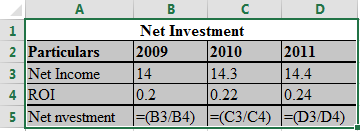The result of the above excel table is as follows: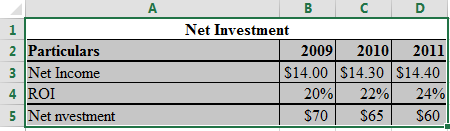Hence, the net investment of the company is \$ Now, calculate the residual income, using MS-excel as given below: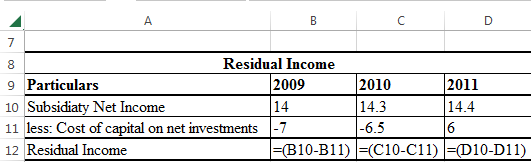The result of the above excel table is as follows: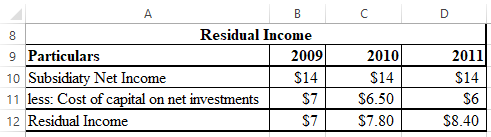The above calculation shows that there is an increase in residual income just as ROI. Thus, it won't be wrong to say that in the case of the subsidiary company, a lot of debt has been added to its capital structure, thereby reducing the net investments.

Variable Costs Variable costs are the costs which change with the change in the level of output. Examples of variable costs comprise of raw material, packaging, etc.Present the full costs transfer pricing methods on P's Cash flow, using excel as given below: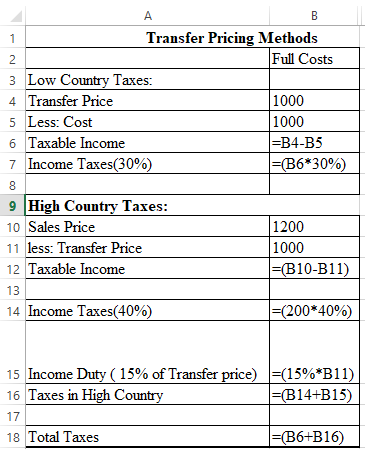The result of the above excel table is as follows: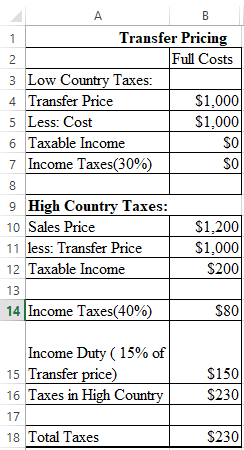Now, present the variable costs transfer pricing methods on P's Cash flow, using excel as given below: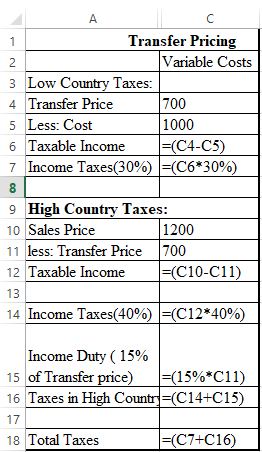The result of the above excel table is as follows: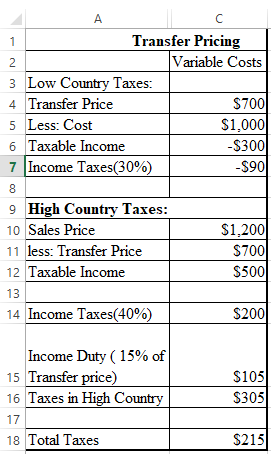In the above calculation, it has been assumed that there is positive taxable income in the low country. This is done so as to offset the loss incurred on transferring loss at certain variable costs then, in that case, variable cost transfer pricing method is used, as it reduces the combined tax liability

Return on Assets It is an indicator of a company's Earnings before Interest and Taxes relative to total net assets. Basically, this ratio reflects, how efficiently a company is utilizing its total assets before executing its prescribed obligations. The higher the ratio, indicates the more favorable scenario for investors, as it reflects that the company is managing its assets proficiently so as to enhance its net income. A positive rate of return on total assets ratio shows an upward profit trend, secondly, it also enables a company in making comparisons with other companies in the same Industry. a.While calculating the ROA, interest has to be excluded, as it is the evaluation of the return on total assets, not the return to the equity investors. Calculate the net income before taxes of S Properties', using excel as shown below: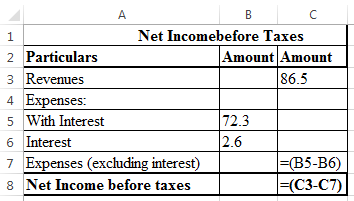The result of the above excel table is shown below: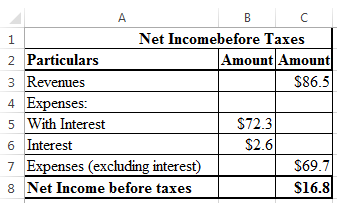Therefore, net income before taxes of S Properties is \$16.8 Calculate the ROA of S Properties' using excel as shown below: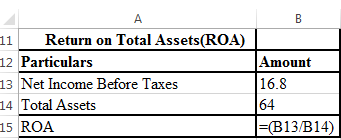The result of the above excel table is shown below: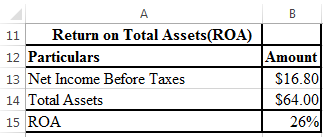Therefore, the ROI of S Properties is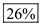b.Calculate the ROA of valley view using excel as shown below: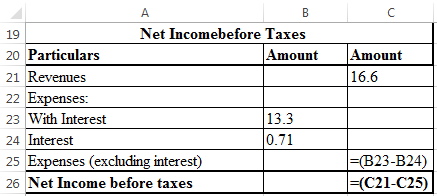The result of the above excel table is shown below: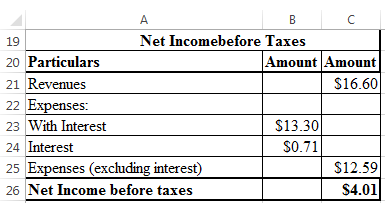Therefore, net income before taxes of valley view is \$4.01 Calculate the ROA of valley view using excel as shown below: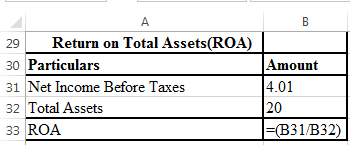The result of the above excel table is shown below: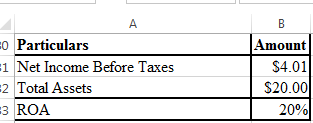Therefore, ROA of Valley view is 20% As the ROA of the valley view is 20% which is quite less than the ROA of the firm, which is 26%. Therefore, Sundars' will be affected if the project of valley view is adopted. Hence, it is advisable for the management to reject the project of valley view. c. The shareholders of B Holdings demand that if Valley View has a positive residual income, the managers of Sundar Properties should definitely purchase the same. Calculate the residual income of the company, using excel as shown below: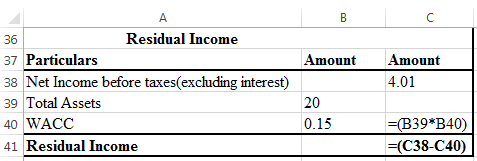The result of the above excel table is shown below: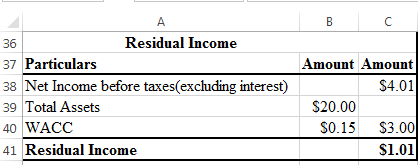Therefore, the residual income of the company is \$1.01 . As through the above calculations, it is seen that the residual income of the company is positive and therefore, the demands of the shareholders shall be fulfilled.Another way to find the reliability of the valley view project is that Valley View has a return on investment of 20% which is beyond the Sundars' weighted average cost of capital of 15%, hence, Valley view is a lucrative acquisition. d.When providing the compensation to the managers of S Properties, on the basis of ROA provides them the incentives to under-invest. As it is seen in part (b), that the managers of Sundar Properties have discarded the valley view project, just because it reduces their average ROa.Brighton holdings need to compensate Sundar Properties management on the basis of residual income and not on the basis of ROa.